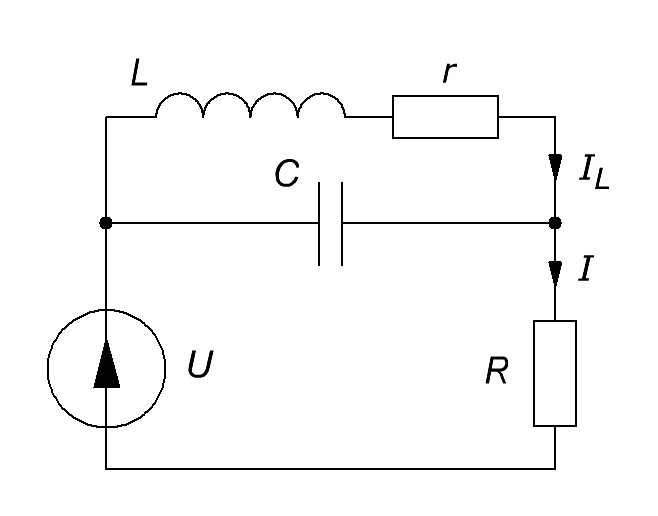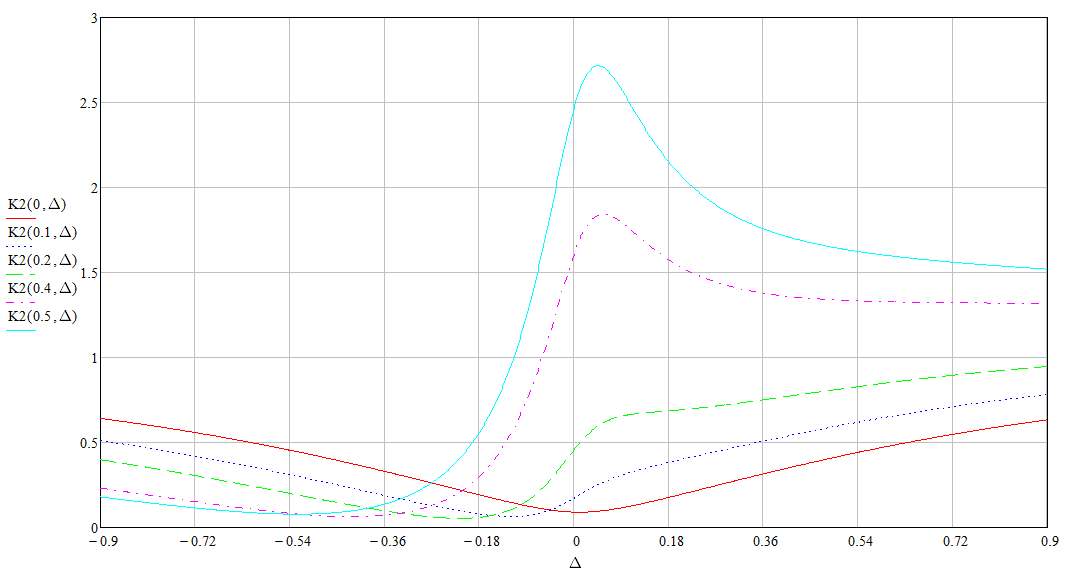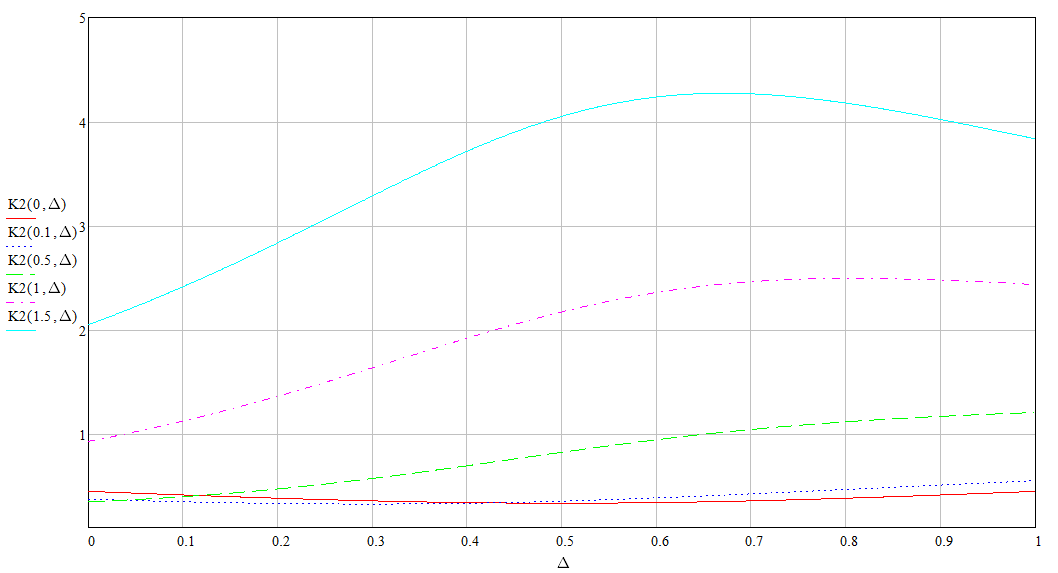Research website of Vyacheslav Gorchilin
2020-06-11
Energy of the phase shift of the current in the inductor
This work is an attempt of theoretical substantiation of the effect, which was obtained as a result of a series of experiments with the phase shift of the current in the inductor. Its meaning is quite simple. In the circuit (Fig. 1) consisting of a source of sinusoidal voltage U, the parallel resonant circuit LC and active resistance R, at some point of time, say every tenth oscillation, shifts the phase of the current $$I_L$$ in the coil L.Fig.1. The scheme to calculate energy phase shift
The rest circuit, in this moment, fails to respond to the rapid change in phase, and the transition process lasts at least ten oscillations, and the chain recovers its initial settings. In fact, we are talking about the effective conversion of reactive power to active.
To obtain such a shift is a parametric method that is described in detail in the methodology of this experiment. Since the receipt of such a shift, likely, possible, and other methods, we present here a mathematical model, though approximate, but the most generalized.
For the perfect solution to this problem would require the use of recursive differential equations that is difficult or impossible without the use of computing, and our goal is to obtain estimates of this effect in the analytical form. Therefore, we apply not the usual method, based on the assumption that in comparison with the rate of change of phase current the rate of change of the amplitude of the current in the coil can be considered slowly varying. This approach is very idealized and does not take into account some parameters, but it is relatively simple and it is possible to apply the classical "Method of complex amplitudes" [1-3].
Alogrithm our actions following. First, you must find the dependence of the current in the load R from the current in the inductance L: $I = a\ U + b\, I_L \qquad (1)$ where: $$a, b$$ — some factors which in General, can be complex; the current in the inductor $$I_L$$ has the complex value, which shows the phase shift relative to the source voltage $$U$$, that these fluctuations are: $I_L = Re(I_L) + \mathbf{i}\, Im(I_L) \qquad (2)$ Here $$Re(I_L), Im(I_L)$$ respectively, the valid and imaginary current value, the ratio between which and gives this phase. But it is a permanent phase shift in a steady process, and we must here take into consideration estimated in the experiment, a quick phase shift, which circuit, according to our assumption, do not have time to react. Accounting for this value in electronics is simple: the current damnaged on Euler's formula $$e^{\mathbf{i} \alpha}$$ , where $$\alpha$$ — angle quick phase shift. The value of the current in the inductance, which takes into account both steady-state and transient value of the phase now would be: $I_{\alpha} = a\ U + b\, I_L\, e^{\mathbf{i} \alpha} \qquad (3)$ to distinguish the steady-state current from the current offset, the last one will be denoted now as $$I_{\alpha}$$. You can see that if the angle $$\alpha$$ is equal to zero, then (3) turns into formula (1). The next step of our algorithm is the calculation of the energy ratios.
Energy phase shift
To calculate the power ratio allocated to nargusta R with a rapid phase shift, we need to compare two power: the power required for the power supply of all circuit and power generated in the load. They are so: $P = |I|\, U, \quad P_{\alpha} = |I_{\alpha}|^2 R \qquad (4)$ Here the currents are taken at the module. This is important. Then increase the efficiency of the second kind will be the relations of these capacities are: $K_{\eta 2} = {P_{\alpha} \over P} = {|I_{\alpha}|^2 R \over |I|\, U} \qquad (5)$ In the formula (5) are not taken into account the cost of the device, creating a phase shift and the usual efficiency of the actual installation. We will do this later when more accurate calculations for real systems. While it is important for us to obtain some qualitative and quantitative parameters to confirm the effect.
Calculation
Use the rule of Kirchhoff  for the circuit (Fig. 1) and by the method of complex amplitudes [1-3] will make two equations: $I\, R = U - I_L\, X_L \qquad (6)$ $I = U {X_L + X_C \over R (X_L + X_C) + X_C X_L} \qquad (7)$ where: $$X_L = \mathbf{i} \omega L + r$$ — complex resistance of the coil inductance L and its resistance $$r$$, and $$X_C = 1 / \mathbf{i} \omega C$$ — the reactance of the capacity C. In these formulas, $$\omega$$ angular frequency . From these formulas, by some transformations, we obtain the following currents: $I = \frac{U}{R} {G \over G + H}, \quad G = \Delta + \mathbf{i} {1 - \Delta \over Q} \qquad (8)$ $I_{\alpha} = \frac{U}{R} {G + H (1 - e^{\mathbf{i} \alpha}) \over G + H}, \quad H = q\, (1/Q + \mathbf{i}) \qquad (9)$ Here: $$Q = \omega L / r$$ — q LC circuit; $$q = \omega L / R$$ is in the quality system; $$\Delta = 1 - \omega^2 LC$$ — the coefficient of variation of the resonance (when the resonance this parameter equal to zero). Now, the obtained formula, we substitute in (5) and derive the value of growth efficiency: $K_{\eta 2} = {|G + H (1 - e^{\mathbf{i} \alpha})|^2 \over |G + H| |G| } \qquad (10)$ As you can see from the last formula, if $$\alpha = 0$$, i.e. the phase shift is not present, and the increase in efficiency cannot exceed one. But let's consider the cases when the phase shift is.
Some patterns
The first thing you can immediately notice if the value of the angle of phase shift is insufficient, the desired effect is not achieved. The following graph (Fig. 2) dependences of the efficiency gains from the phase angle $$\alpha$$ and the coefficient of variation of the resonance $$\Delta$$ (KOR). Here you can see that if $$\alpha = 0$$ (red graph), the growth efficiency is always less than unity. When you increase the shear angle, after a certain value, the increase is beyond a single value, which could mean, in practice, the appearance of the desired effect. In this case, this occurs when $$\alpha \gt 0.25$$. For example, a crimson dotted curve in $$\alpha = 0.4$$, reaches maximum values of 1.8.
Second, the graph shows that the maximum gain is not exactly in resonance, and slightly above it. You can check that if the shear angle is negative, then this maximum will be located below the resonance. This was observed in the experiment.Fig.2. The dependence of the growth efficiency of the shear angle and the COR at Q = 10, q = 1
This graph (Fig. 2) is built with the following q-switch: $$Q = 10, q = 1$$, but let's change these parameters to other, for example, increase the q of the circuit 100. As you can see (Fig. 3), the gain also increased, but the minimum value of the phase angle at which the effect appears, still remain.Fig.3. The dependence of the growth efficiency of the shear angle and the COR at Q = 100, q = 1
Let's increase to ten and also the q of the system, $$q$$, which will significantly increase the maximum values of growth efficiency (Fig. 4).Fig.4. The dependence of the growth efficiency of the shear angle and the COR at Q = 100, q = 10
But is it possible to get the effect in both q-switch is equal to one? According to the math — you can, however it will have to increase the oscillator frequency is approximately 1.3 times the resonant frequency of the circuit, and the phase angle to be made large enough (Fig. 5). By the way, the angle in this work, everywhere is calculated, in radians.Fig.5. The dependence of the growth efficiency of the shear angle and the COR at Q = 1, q = 1
Some simplifications
Formula (10), on which was built all the graphics are fairly bulky. But if you accept some conditions, it can be great to simplify. If we sample that the system is in resonance, the shear angle is small, and q on the contrary is large enough, then (10) simplifies as $K_{\eta 2} \approx q\, Q\, \alpha^2, \quad \Delta = 0, \quad q\, Q\, \alpha \gg 1, \quad \alpha \lt 0.45 \qquad (11)$
Example according to the formula (11)
Suppose that the oscillator frequency of 16 kHz, the inductance of the coil L = 1 mH, the load R = 100 Ω, and the shear angle $$\alpha = 0.12$$. Then, from formula (11) it follows that for increase of efficiency of more than units it is required that the product of two q-switch the $$q \gt 70$$. The coil impedance at the frequency of the oscillator: $$\omega L = 100$$ Ohms, so the q of the system is equal to one: $$q = 1$$. Hence, it is necessary to increase the coil quality factor $$Q$$ at least until 70, and taking into account the losses in the efficiency of such transformation and the shift, this parameter should be increased many times more. In reality, this can be achieved by applying a thick copper wire, ideally made of oxygen-free copper.
Also, it is possible to calculate the duration of the shift. Given that the angle is considered in radians, the full period will be equal to $$2 \pi$$, the duration of the shift will last for approximately 2% of the time from the complete vibration generator, or by time of approximately 1 µs.
Insights
In this work it was presented a possible theoretical justification for experimentally obtained effect of the phase shift of the current in the inductor. In the formulas derived here not taken into account some parameters, such as dielectric loss of a capacitor or wires. Also not taken into account the efficiency of the elements of the generator and the cost of creating a phase shift. However, in a certain approximation it was possible to identify and the effect itself and some patterns in mathematical analytical form, which can be a platform for further development of this undoubtedly interesting topic.
From the graphs presented in this work clearly shows different curve classic resonance and resonance when the phase shift of the current maximum shifts depending on the shift angle. This point will need to consider when razrabotke devices operating on this principle.
There are some features of the application derived in this work formulas. For example, in the beginning, we assumed that the current shift will be done only every tenth oscillation. It follows that the average growth rate of efficiency, you must divide by 10. Since the response time of a LC-resonator is determined by its q-factor and q-factor is approximately equal to the number of vibrations, in the more General case, the obtained results correct to divide the parameter $$Q$$. At the same time, the number of oscillations, through which the phase shift also approximately equal to $$Q$$. Despite this caveat, the increase in efficiency is greater than one are possible; examples are in figures (4) and (5).
Hence it follows directly that for maximum effect you need to increase not so much the quality factor of the LC-circuit, as the q of the system, $$q$$. Also, real devices must achieve a minimum phase angle of the current in which is observed the effect.
The materials used
1. L. A. Bessonov. Theoretical foundations of electrical engineering. 1996. – 638 C, PP 3.11. Basis symbolic method for calculating circuits with sinusoidal current.
2. The use of complex numbers for calculation of electric circuits (Method of complex amplitudes).
3. LECTURE 4. The method of complex amplitudes.
4. Wikipedia. The Formula Of Euler.
5. Wikipedia. Rules Of Kirchhoff.
6. Wikipedia. Angular frequency.